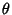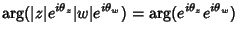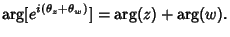## Argument (Complex Number)

A Complex Numbermay be represented as(1)

whereis called the Modulus of, andis called the argument(2)

Therefore,(3)

Extending this procedure gives(4)

The argument of a Complex Number is sometimes called the Phase.

See also Affix, Complex Number, de Moivre's Identity, Euler Formula, Modulus (Complex Number), Phase, Phasor

References

Abramowitz, M. and Stegun, C. A. (Eds.). Handbook of Mathematical Functions with Formulas, Graphs, and Mathematical Tables, 9th printing. New York: Dover, p. 16, 1972.